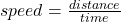## How long does it take for a short pulse of light to travel from one end of the glass to the other? Express your answer in terms of the frequ

Question

How long does it take for a short pulse of light to travel from one end of the glass to the other? Express your answer in terms of the frequency, f. Use the numeric value given for n in the introduction.

in progress 0
3 months 2021-08-06T10:07:17+00:00 1 Answers 29 views 0

1. Complete Question:

A beam of light from a monochromatic laser shines into a piece of glass. The glass has thickness Land index of refraction n=1.5. The wavelength of the laser light in vacuum is L/10 and its frequency is f. In this problem, neither the constant c nor its numerical value should appear in any of your answers.

How long does it take for a short pulse of light to travel from one end of the glass to the other?

Express your answer in terms of the frequency, f. Use the numeric value given for n in the introduction.

15/f

Explanation:

Wavelength of the laser light, λ = L/10

Where L = Distance covered by the light

Refractive index, n = 1.5

Speed, v = λf

Since we are considering the laser light, the speed of light, c, will be used.

c = v

c = λf

c = (L/10)f(L/10)f = L/t

f/10 = 1/t

t = 10/f

since the refractive index, n = 1.5

t = 1.5(10/f)

t = 15/f